StatLect

Probability density function

The distribution of a continuous random variable can be characterized through its probability density function (pdf). The probability that a continuous random variable takes a value in a given interval is equal to the integral of its probability density function over that interval, which in turn is equal to the area of the region in the xy-plane bounded by the x-axis, the pdf and the vertical lines corresponding to the boundaries of the interval.

For example, in the picture below the blue line is the pdf of a normal random variable and the area of the red region is equal to the probability that the random variable takes a value comprised between -2 and 2.Definition

The following is a formal definition.

Definition The probability density function of a continuous random variableis a function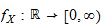such that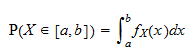for any interval.

The set of valuesfor whichis called the support of.

Example

Suppose that a random variablehas probability density functionTo compute the probability thattakes a value in the interval, you need to integrate the probability density function over that interval:The probability density is not a probability

It is important to understand a fundamental difference between the probability density function, which characterizes the distribution of a continuous random variable, and the probability mass function, which characterizes the distribution of a discrete random variable (remember: a random variable is discrete if the number of values it can take is countable, while the number of values that a continuous random variable can take is uncountable). The probability mass function of a discrete variableis a functionthat gives you, for any real number, the probability thatwill be equal to. On the contrary, ifis a continuous variable, its probability density functionevaluated at a given pointis not the probability thatwill be equal to. As a matter of fact, this probability is equal to zero for anybecause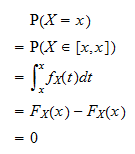whereis any primitive (or indefinite integral) of.

If you are puzzled by the latter result, you are advised to read the lecture on zero-probability events.

Although it is not a probability, the value of the pdf at a given pointcan be given a straightforward interpretation: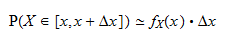whereis a small increment.

Proof

The proof we are going to give is not rigorous. Rather, we are focusing on the intuition. For the sake of simplicity, we assume that the pdf is a continuous function. Strictly speaking, this is not necessary, although most of the pdfs that are encountered in practice are continuous (by definition, a pdf must be integrable; however, while all continuous functions are integrable, not all integrable functions are continuous). If the pdf is continuous andis small, thenis well approximated byfor anybelonging to the interval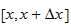. It follows that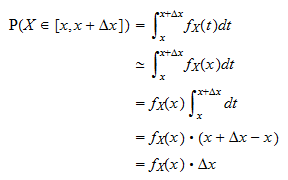In the above approximate equality, we consider the probability thatwill be equal toor to a value belonging to a small interval near. In particular, we consider the interval. The probability is proportional to the lengthof the small interval we are considering. The constant of proportionalityis the probability density function ofevaluated at. Thus, the higher the pdfis at a given point, the higher is the probability thatwill take a value near.

Related concepts

Related concepts are those of:

More details

Probability density functions are discussed in more detail in the lecture entitled Random variables.